## 建模前，part 1

def read_and_decode(filename, batch_size):
# 建立文件名隊列
filename_queue = tf.train.string_input_producer([filename],
num_epochs=None)

# 數據讀取器

# 數據解析
img_features = tf.parse_single_example(
serialized_example,
features={ 'Label'    : tf.FixedLenFeature([], tf.int64),
'image_raw': tf.FixedLenFeature([], tf.string), })

image = tf.decode_raw(img_features['image_raw'], tf.uint8)
image = tf.reshape(image, [42, 42])

label = tf.cast(img_features['Label'], tf.int64)

# 依序批次輸出 / 隨機批次輸出
# tf.train.batch / tf.train.shuffle_batch
image_batch, label_batch =tf.train.shuffle_batch(
[image, label],
batch_size=batch_size,
capacity=10000 + 3 * batch_size,
min_after_dequeue=1000)

return image_batch, label_batch

# tf.train.shuffle_batch 重要參數說明
# tensors：   排列的張量。
# batch_size：從隊列中提取新的批量大小。
# capacity：  一個整數。隊列中元素的最大數量。
# min_after_dequeue：出隊後隊列中的最小數量元素，用於確保元素的混合級別。


Batch_Size 是機器學習中一個重要的參數，Batch 的選擇會決定梯度下降的方向。

## 建模前，part 2

### 訓練集樣本不平衡的問題。

The Impact of Imbalanced Training Data for Convolutional Neural Networks

CIFAR-10是一個簡單的圖像分類數據集。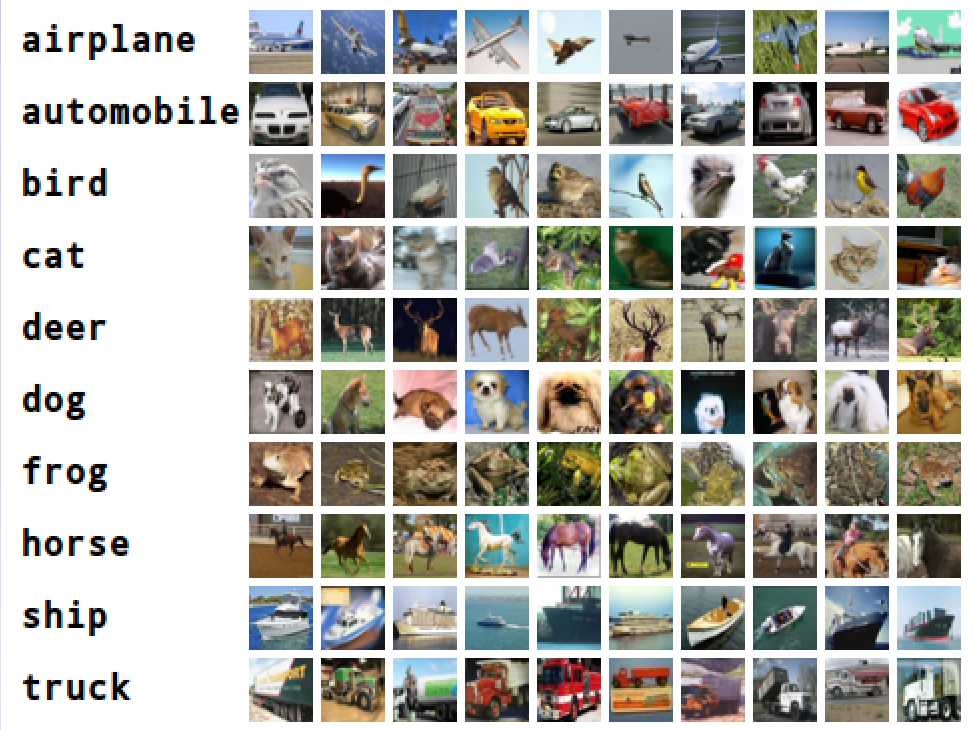圖片來源：CNN训练Cifar-10技巧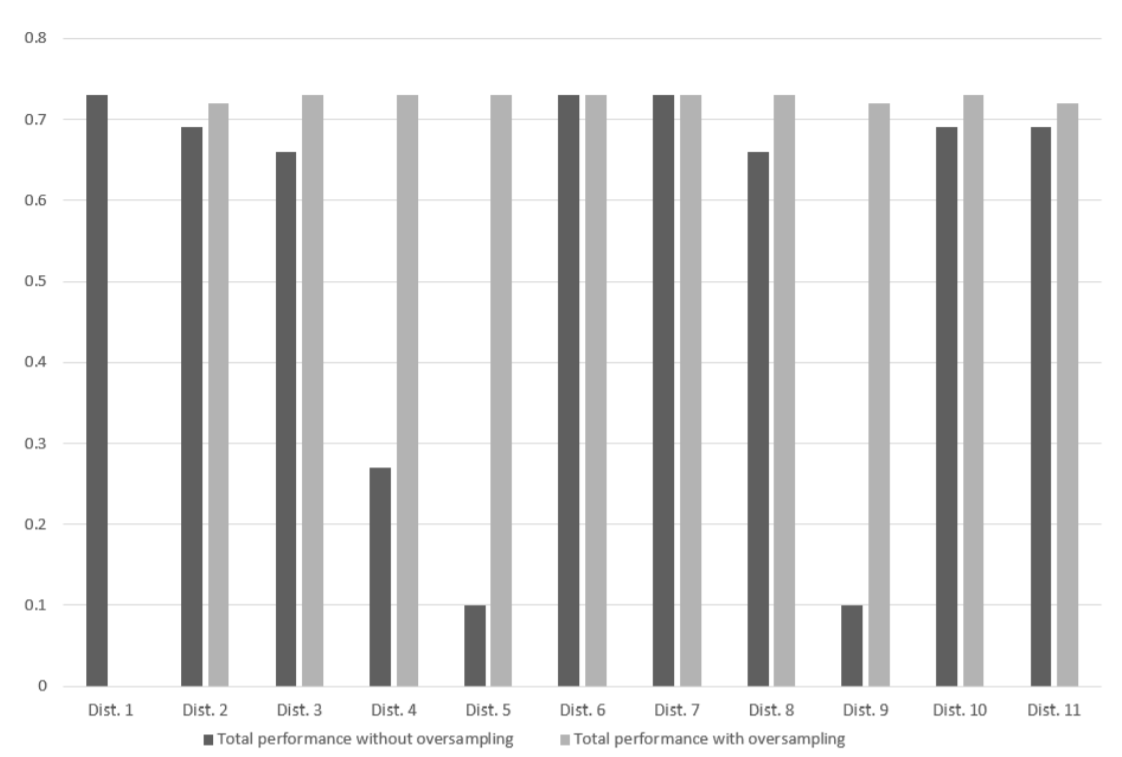Dist. 1：類別平衡，每一類都佔用10%的數據。
Dist. 2、Dist. 3：一部分類別的數據比另一部分多。
Dist. 4、Dist 5：只有一類數據比較多。
Dist. 6、Dist 7：只有一類數據比較少。
Dist. 8： 數據個數呈線性分佈。
Dist. 9：數據個數呈指數級分佈。
Dist. 10、Dist. 11：交通工具對應的類別中的樣本數都比動物的多。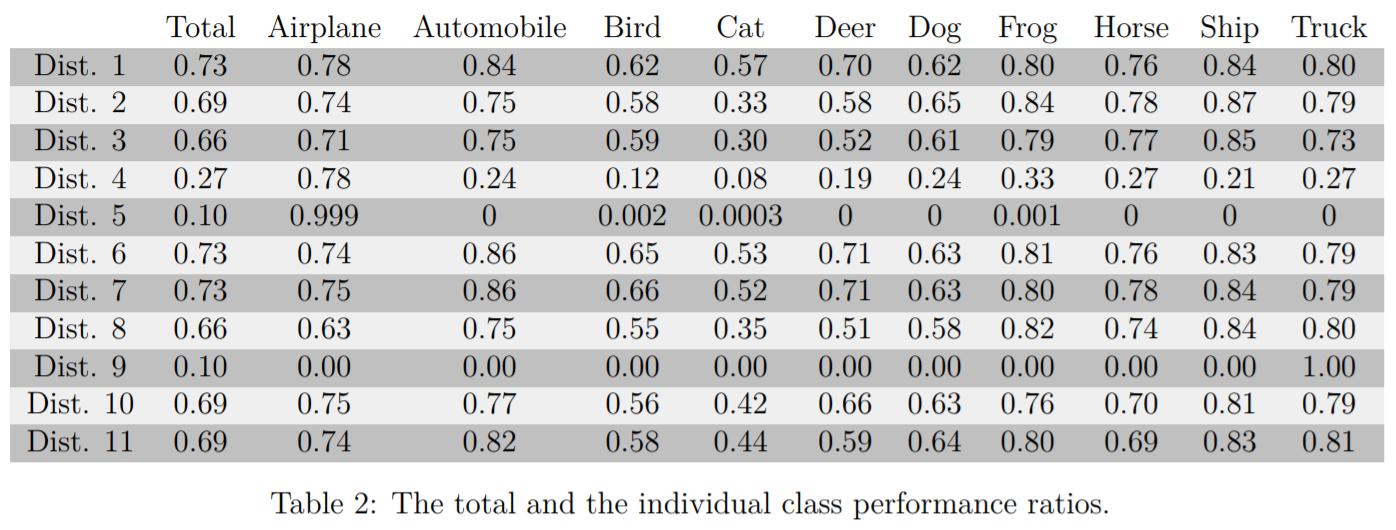1. 類別完全平衡時，結果最好。
2. 類別愈是扭曲，效果越差。

import os
import numpy as np

def get_File(file_dir):
# The images in each subfolder
images = []
# The subfolders
subfolders = []

# Using "os.walk" function to grab all the files in each folder
for dirPath, dirNames, fileNames in os.walk(file_dir):
for name in fileNames:
images.append(os.path.join(dirPath, name))

for name in dirNames:
subfolders.append(os.path.join(dirPath, name))

# To record the labels of the image dataset
labels = []
count = 0
for a_folder in subfolders:
n_img = len(os.listdir(a_folder))
labels = np.append(labels, n_img * [count])
count+=1

subfolders = np.array([images, labels])
subfolders = subfolders.transpose()

image_list = list(subfolders[:, 0])
label_list = list(subfolders[:, 1])
label_list = [int(float(i)) for i in label_list]
return image_list, label_list

1. 自動計算所有類別內的圖檔數量。
2. 以最低圖檔數的類別為基準，從每個類別隨機挑選出基準量的圖檔。

import os
import random as r
import numpy as np

def get_File(file_dir):

# The images in each subfolder
images = []

# The subfolders
subfolders = []

# Using "os.walk" function to grab all the files in each folder
for dirPath, dirNames, fileNames in os.walk(file_dir):

names = []
for name in fileNames:
names.append(os.path.join(dirPath, name))

for name in dirNames:
subfolders.append(os.path.join(dirPath, name))

# 隨機打亂各個資料夾內的數據
r.shuffle(names)
if names != []:
images.append(names)

# 計算最小檔案數量的資料夾
mincount = float("Inf")
for num_folder in subfolders:
n_img = len(os.listdir(num_folder))

if n_img < mincount:
mincount = n_img

# 只保留最小檔案數量
for i in range(len(images)):
images[i] = images[i][0:mincount]

images = np.reshape(images, [mincount*len(subfolders), ])

# To record the labels of the image dataset
labels = []
for count in range(len(subfolders)):
labels = np.append(labels, mincount * [count])

# 打亂最後輸出的順序，去除每個類別間的隔閡
subfolders = np.array([images, labels])
subfolders = subfolders[:, np.random.permutation(subfolders.shape)].T

image_list = list(subfolders[:, 0])
label_list = list(subfolders[:, 1])
label_list = [int(float(i)) for i in label_list]
return image_list, label_list

OK，到此為止，複習完畢！

## 建一個簡單的字元識別模型

Softmax 函數在數學，尤其是機率論和相關領域中，Softmax函數，或稱歸一化指數函數，是邏輯函數的一種推廣。它能將一個含任意實數的 K 維向量「壓縮」到另一個K維實向量中，使得每一個元素的範圍都在 (0,1) 之間，並且所有元素的和為1。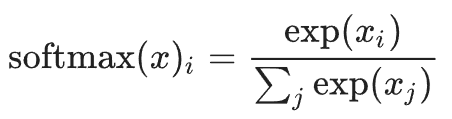import math

z = [1.0, 2.0, 3.0, 4.0, 1.0, 2.0, 3.0]
z_exp = [math.exp(i) for i in z]

print(z_exp)
# Result: [2.72, 7.39, 20.09, 54.6, 2.72, 7.39, 20.09]

sum_z_exp = sum(z_exp)
print(sum_z_exp)
# Result: 114.98

softmax = [round(i / sum_z_exp, 3) for i in z_exp]
print(softmax)
# Result: [0.024, 0.064, 0.175, 0.475, 0.024, 0.064, 0.175]

Softmax 函數資料來源：維基百科，Softmax函數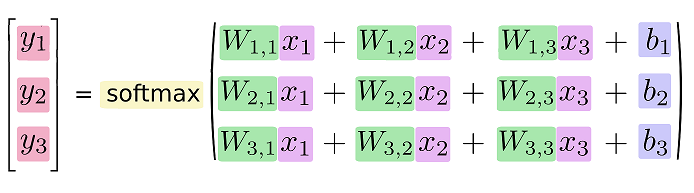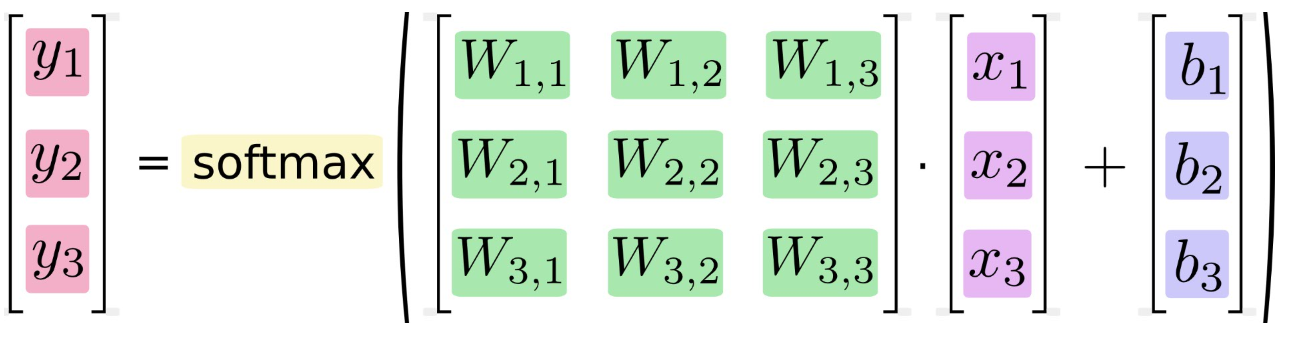圖片來源：https://www.tensorflow.org/versions/r1.2/get_started/mnist/beginners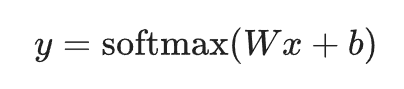# -*- coding: utf-8 -*-
"""

"""

import tensorflow as tf

# 建立文件名隊列
filename_queue = tf.train.string_input_producer([filename], num_epochs=None)

# 數據讀取器

# 數據解析
img_features = tf.parse_single_example(
serialized_example,
features={ 'Label'    : tf.FixedLenFeature([], tf.int64),
'image_raw': tf.FixedLenFeature([], tf.string), })

image = tf.decode_raw(img_features['image_raw'], tf.uint8)
image = tf.reshape(image, [42, 42])

label = tf.cast(img_features['Label'], tf.int64)

# 依序批次輸出 / 隨機批次輸出
# tf.train.batch / tf.train.shuffle_batch
image_batch, label_batch =tf.train.shuffle_batch(
[image, label],
batch_size=batch_size,
capacity=10000 + 3 * batch_size,
min_after_dequeue=1000)

return image_batch, label_batch

# 自己做好的 TF 檔在哪裡，自己知道
filename = './py_Train.tfrecords'

# batch 可以自由設定
batch_size = 256

# 0-9共10個類別，請根據自己的資料修改
Label_size = 10

# 調用剛才的函數

# 轉換陣列的形狀
image_batch_train = tf.reshape(image_batch, [-1, 42*42])

# 把 Label 轉換成獨熱編碼
label_batch_train = tf.one_hot(label_batch, Label_size)

# W 和 b 就是我們要訓練的對象
W = tf.Variable(tf.zeros([42*42, Label_size]))
b = tf.Variable(tf.zeros([Label_size]))

# 我們的影像資料，會透過 x 變數來輸入
x = tf.placeholder(tf.float32, [None, 42*42])

# 這是參數預測的結果
y = tf.nn.softmax(tf.matmul(x, W) + b)

# 這是每張影像的正確標籤
y_ = tf.placeholder(tf.float32, [None, 10])

# 計算最小交叉熵
cross_entropy = tf.reduce_mean(tf.nn.softmax_cross_entropy_with_logits_v2(labels=y_, logits=y))

# 使用梯度下降法來找最佳解

# 計算預測正確率
correct_prediction = tf.equal(tf.argmax(y, 1), tf.argmax(y_, 1))
accuracy = tf.reduce_mean(tf.cast(correct_prediction, tf.float32))

with tf.Session() as sess:
# 初始化是必要的動作
sess.run(tf.global_variables_initializer())
sess.run(tf.local_variables_initializer())

# 建立執行緒協調器
coord = tf.train.Coordinator()

# 啟動文件隊列，開始讀取文件

# 迭代 10000 次，看看訓練的成果
for count in range(10000):
# 這邊開始讀取資料
image_data, label_data = sess.run([image_batch_train, label_batch_train])

# 送資料進去訓練
sess.run(train_step, feed_dict={x: image_data, y_: label_data})

# 這裡是結果展示區，每 10 次迭代後，把最新的正確率顯示出來
if count % 10 == 0:
train_accuracy = accuracy.eval(feed_dict={x: image_data, y_: label_data})
print('Iter %d, accuracy %4.2f%%' % (count, train_accuracy*100))

# 結束後記得把文件名隊列關掉
coord.request_stop()
coord.join(threads)

【Python】TensorFlow學習筆記(五)：存檔 & 讀檔